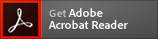This site requires the use of
If you need to upgrade click the
icon below.Ianik Plante
4/1/2021

# Abstract

In radiobiology, many dose-response results are modeled using the so-called linear-quadratic (LQ) model, which means that results are modeled as a function of dose D as R(D)=β01D+β2D2. The coefficients β0, β1 and β2 are obtained from fitting a series of data points (xi,yi), which is usually done using a least-square method. The LQ and more generally the polynomial fit capability is implemented in many software that analyzes data. However, there are some instances where the fitting needs to be done programmatically. Furthermore, depending on the software used, some features may not be implemented. In this mini-review, I discuss the basis of polynomial fitting, including the calculation of errors on the coefficients and results, use of weighting and fixing the intercept value (the coefficient β0). A simple C++ code to perform the polynomial curve fitting is also provided. This code should be useful not only in radiobiology but in other fields of science as well.